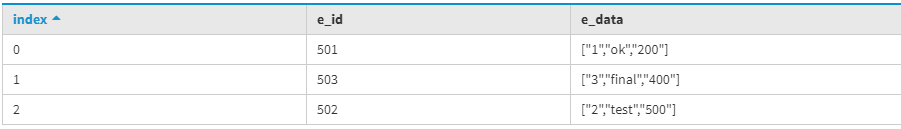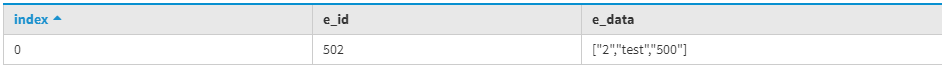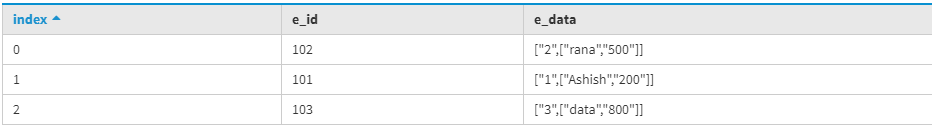Tuple Type in Cassandra

• Last Updated : 05 Dec, 2019

In this article we will discuss Tuple Data Type which is supported by Cassandra Query Language in which we can easily defined data as per need. It is an alternative to a User Defined Data Type.

A tuple data type is useful when we need to accommodate many fields we can accommodate maximum 32768 fields. let’s understand tuple data type with an example.

Here, first we are going to create table for example Emp_data is a table name and E_id is primary key of table and E_data field is tuple type in below given table. let’s have a look.

CREATE TABLE Emp_data (
E_id int PRIMARY KEY,
E_data tuple<int, text, int>
);

Now, we are going to insert some data in Emp_data table by using following CQL query.

INSERT INTO Emp_data (E_id, E_data)
VALUES(501, (1, 'ok', 200));

INSERT INTO Emp_data (E_id, E_data)
VALUES(502, (2, 'test', 500));

INSERT INTO Emp_data (E_id, E_data)
VALUES(503, (3, 'final', 400));

Now, to see the output Emp_data table used the following CQL query.

SELECT * FROM Emp_data;

Output:Now, to apply filter we are going to create indexing on E_data field which is tuple data type.

CREATE INDEX on Emp_data (E_data);

We can used tuple type field in where clause to filter data.

SELECT *
FROM Emp_data
WHERE E_data = (2, 'test', 500);

Output:Tuple type can be used for nested purpose also. Again we are going to create a new table for nested purpose.so, let’s have a look.

CREATE TABLE nested_Emp_data
(
E_id int PRIMARY KEY,
E_data tuple<int, tuple< text, int>>
);

Now, insert data into nested_Emp_data tableby using following CQL query.

INSERT INTO nested_Emp_data (E_id, E_data)
VALUES (101, (1, ('Ashish', 200)));

INSERT INTO nested_Emp_data (E_id, E_data)
VALUES (102, (2, ('rana', 500)));

INSERT INTO nested_Emp_data (E_id, E_data)
VALUES (103, (3, ('data', 800)));

To see the output used the following CQL query.

select *
from nested_Emp_data;My Personal Notes arrow_drop_up